# The Independence GameSubscribe!My latest posts can be found here:
Previous blog posts:
Additionally, some earlier writings:

## The Independence Game - 2017/04/16

 This page has been Tagged As Maths.
A few weeks ago I was honoured to be able to attend the gathering at the Royal Society where Rob Eastaway was given his Christopher Zeeman medal. He gave an excellent talk, full of interest and humour, the only downside being that he made us play a game. I don't remember if he gave it a name (Update: Rob calls it "Avoid the Neighbours") but I now think of it simply as "Rob's Dots". He said he didn't know how to play it optimally beyond 11 points, so I thought I'd have a go.

It goes like this:

 Starting position:Player one marks a dot:which eliminates its neighbours:Player 2 marks another dot:leaving player one to take a dot:and win!
You have a row of dots, and you and your opponent take it in turns to mark one of them. The rule is that you can't mark a neighbour of an already marked dot, and if you thereby can't move, you lose.

Here at right is an example game with 6 dots. Player 1 takes a dot somewhere in the middle, and that means now neither player can take the dot on either side. Player 2 removes the left-most dot, leaving the two on the far right.

Player 1 then wins by taking one of the remaining dots, which also eliminates the other, because it's a neighbour. So in this example game, Player 1 wins.

Now suppose we start with an odd number of dots. If Player one marks the middle dot, removing it and its neighbours from the game, we are left with dots on the left, and dots on the right, and no way of interacting. Now no matter what Player 2 does on one side, Player 1 can copy on the other. As a result Player 1 is guaranteed always to have a move, and therefore is guaranteed to win.

So with an odd number of dots, Player 1 can always win by marking the middle dot. (There might be other winning moves, but, well, who cares?)

So any row with an odd number of dots is a win for the first player.

Starting from scratch, a row with just 1 or 2 dots is a win for the first player, because they mark it, and that's the end. A row of 3 dots is a win for the first player if they choose the middle dot. If they are foolish enough to choose an end dot then the second player still has a place to play, takes it, and wins.

On the other hand, a row of 4 dots is a win for the second player, I'll leave it to the (mythical) interested reader to work that out, and to work out whether the 6 dot game is a win for the first or second player.

## First digression, a generalisation

You can skip this section if you want to - we're going to do a little Graph Theory.

A "Graph" or "Network" is a collection of nodes (also called vertices), some of which are joined by edges (also called lines).

Two vertices joined by an edge are called "Adjacent" or "Neighbours".

A set S of vertices is called "Independent" if it doesn't contain a pair of adjacent vertices. S is also called "Maximal" if every vertex is either already in the set, or has a neighbour who is. So once you have a maximal independent set of vertices in a graph, you can't add any more.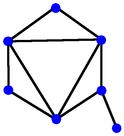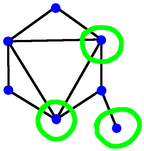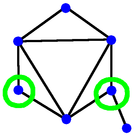A graph Not independent Not maximally independent

So we could play a game. Pick a graph, then we take it in turns to pick a vertex to add to an independent set. First one who can't do that wins. Played on a general graph this game is called "Monochrome".Maximal independent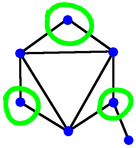Maximal independent
And with all that, we get this:

 Rob's Dots game is "Monochrome" where the chosen graph is a path.

I'm glad we got that settled. Moving on ...

### An alternate digression - misère

There is another variation that we might consider in which the last player to move loses instead of wins. This is called the misère variant, and in general is much, much harder to analyse. We will ignore that here, but it's a fascinating and challenging area.

## Second digression, the game of Nim

Now let me introduce you, or perhaps re-introduce you, to the game of Nim. You may have met it before, but bear with me - this is relevant.

Very relevant.

In Nim we have some heaps of tokens - the heaps need not all be of the same size, and in general, aren't. On each move a player may take any number of items from any single heap, including the option of taking an entire heap. As with the dots game, if you can't make a move, then you lose.

 The game of Nim has been solved. What does that mean? For a game to be solved means that given a game position there is a simple (or sometimes not so simple) calculation one can make to determine whether it is a win for player 1 or player 2. If it's a win for Player 1, and it's your turn, that means there is a winning move. So you then can look at all the positions you can move to and pick one that is a win for Player 2. Then do that.
There are some similarities here between Nim and the dots game. We can look at the collection of dots as starting as a single component, and then when you mark a dot, and thereby eliminate its neighbours, you've broken up the remaining dots into separate collections. We call them components, because when we think of this as an example of "Monochrome", taking vertices can separate the graph into separate pieces, and the separate pieces of graphs are called components.

So in the dots game, whenever there are two components of the same size (and more generally, in a game of Monochrome when there are two components that are isomorphic), whatever one player does in one, the other can mimic in the other, and so it's a second player win. Likewise in Nim, if there are two heaps of equal size then the second player can mimic the first player's moves and win.

So Nim has some similarities to the Dots game, and perhaps things we learn in the study of Nim can be of use in the study of the Dots game. And indeed, as it happens, both are examples of a more general type of game called an Octal Game.

## Converting Dots to Heaps.

Since Nim is well studied and has lots of cousins, there might be value in thinking of the Dots game somehow as a collection of heaps. We do that as follows.

Given that we start with N dots, we think of that as equivalent to a heap with N tokens. When we mark a dot we remove it and any neighbours, and that might leave nothing, one path, or two paths.

So:

• If N=1 or N=2 then the only thing we can do is take the entire heap.

• If N=3 then we can take an endpoint, leaving one dot, or the middle dot, leaving nothing.
• Thus if we have a heap of 3 we can:
• take two items leaving 1, or
• take all of it.

• If N=4 then we can take an endpoint, leaving two dots, or a "middle" dot, leaving 1 dot.
• Thus if we have a heap of 4 we can:
• take two items leaving 2, or
• take 3, leaving 1.

• If N>=5 then we can:
• Take two, leaving a single heap with N-2;
• Take three, leaving a single heap with N-3;
• Take three, and divide the remaining N-3 into two heaps.

 Why is it 0.137 ?? It's explained in the Wikipedia page about Octal Games, but briefly it goes like this. The first digit says what you're allowed to do if you take one token from a heap. You're allowed to: Leave nothing - yes; Leave a single non-empty heap - no; Leave two heaps - no. So that's encoded as the binary number 001, which is 1. The second digit says what you're allowed to do if you take two tokens from a heap. You're allowed to: Leave nothing - yes; Leave a single non-empty heap - yes; Leave two heaps - no. So that's encoded as the binary number 011, which is 3. And so on. The numbers we obtain are then concatenated and thought of as an octal number, since the digits can only range from 0 to 7. Read the Wikipedia page for more details.
You can see how these operations on the heaps correspond to the various options with the path of dots.

This exactly falls into the class of Octal Games, and is given the octal encoding of 0.137. It's been studied a great deal, and is equivalent to Dawson's Chess, a simplified version of which is called Hexapawn, and was written about by Martin Gardner.

(This is one of the advantages of having a consistent encoding of games based on what the game is, rather than just a random name that someone made up. Calling the game "0.137" means we can find other games that are, underneath, the same).

## An analysis of 0.137 ...

So we can now look at the octal game 0.137 and see what we can learn.

A little notation. We can describe a game position by listing the sizes of the heaps. Further, we can put the sizes in order. So an example position might be (2,4,7). The position with no heaps is (), and is a second person win, because the first player has no moves.

 The notation P position means "Previous Player Win". The above comment about Nim being solved means that there is a simple way to determine whether a position is a P position or not, without having to go through the tedious process of investigating the tree of options. Positions that are not P positions are called N positions, for "Next Player Win".
We say that any position which is a second person win is a P position. Thus () is a P position. The earlier analysis also shows that (n,n) is a P position for any (positive integer) value n. It's also easy enough to verify that (4) is a P position.

We can also observe that if we have one P position, say (2,2), and another P position, say (4), then the combination of both sets of heaps, in this case (2,2,4), is also a P position. We capture this idea by saying that a P position is "decomposable" or "reducible" if it's a combination of two or more other, non-trivial P positions, otherwise it's "irreducible". So (2,2,4) is reducible.

Our challenge now is to find all the irreducible P positions for 0.137 ...

## A list of P positions ...

So we already know that (), (4), and (n,n) for every integer value of n>0 are all P positions. What other P positions are there? If we write a computer program we can quickly check that these are the one-heap P positions up to 100:

• 4, 8, 14, 20, 24, 28, 34, 38, 42, 54, 58, 62, 72, 76, 88, 92, 96
• (just writing the size of the heap)

Here are all the two heap irreducible P positions with total number of tokens up to 47:P positions with 2 heaps, each heap <= 50

• (1,2), (1,6), (1,7), (2,6), (2,7), (6,7), (3,11), (5,9), (3,12), (5,10), (3,16), (9,10), (3,17), (1,21), (11,12), (1,22), (2,21), (5,18), (2,22), (5,19), (11,16), (1,26), (6,21), (9,18), (10,18), (11,17), (12,16), (1,27), (2,26), (3,25), (5,23), (6,22), (7,21), (9,19), (10,19), (12,17), (2,27), (7,22), (6,26), (9,23), (10,23), (16,17), (6,27), (7,26), (3,31), (7,27), (11,25), (1,35), (12,25), (1,36), (18,19), (2,35), (2,36), (3,37), (1,40), (16,25), (18,23), (6,35), (11,31), (13,29), (1,41), (17,25), (19,23), (2,40), (6,36), (7,35), (12,31), (21,22), (2,41), (7,36), (5,39), (15,30), (13,33), (6,40), (16,31), (21,26), (6,41), (7,40)

(These are ordered by the total number of tokens).

One thing we might ask is whether the density of P positions settles to some sort of limit. From the chart at right here the 2-heap P positions seem fairly uniformly spread. Does that pattern continue?

But here is a simple observation. A heap with one token is, as far as game play is concerned, exactly the same as a heap with two tokens. In each case the player would have to take the entire heap. So in this sense, 1=2.

That means that every time there is a P position with a 2 in it, we can replace the 2 with a 1. So we can see we have (1,6) and (2,6). We also have (1,7) and (2,7). And then we notice that we also have (6,7). So we start to wonder, if we have (a,b) and (b,c), do we then also have (a,c) ??

Actually, it appears that we do. We have (3,11) and (11,25), and yes, we also have (3,25). We also have (3,3), (11,11), (25,25), and so on. Perhaps we can, in some way, think of 3, 11, and 25 as being equivalent.

For example, although I haven't listed the triples here, there is a triple (2,10,17) and we also have (7,10,17). So remembering that (2,7) is a P position, we might hazard the guess that two numbers a and b such that (a,b) is a P position are, in terms of P positions, "equal" or "equivalent" to each other.

Looking at the pairings in two-heap P positions we find we get the following equivalence classes:

• 1, 2, 6, 7, 21, 22, 26, 27, 35, 36, 40, 41, 55, 56, 60, 61, 69, 70, 74, 75, 89, 90, 94, 95
• 3, 11, 12, 16, 17, 25, 31, 37, 45, 46, 51, 59, 71, 79, 80, 93
• 5, 9, 10, 18, 19, 23, 39, 43, 44, 52, 53, 57, 65, 73, 77, 78, 86, 87, 91
• 13, 29, 33, 47, 48, 63, 67, 81, 82
• 15, 30, 49, 50, 64, 83, 84
• 32, 66

We can start to use this idea with mixed size tuples. So, for example, we can show that (3,5,6) is a P position, so perhaps every P position that has a 3 in it can have that 3 replaced by 5 and 6. So we have a look and find that (3,16) is a P position. Is (5,6,16) a P position?

Yes.

Excellent! Let's try another one. Again, let's find a 3 and replace it with (5,6). We look through and find that (3,7,9) is a P position, so we would hope that (5,6,7,9) would also be. But we look through our program output and don't find it. Has it gone wrong?

No, it hasn't, because (5,6,7,9) is reducible, being made up of (5,9) and (6,7). So it is a P position, and the idea has in fact worked in this example as well.

So whenever we have a triple tuple (a,b,c) it would appear that (a) is equivalent to (b,c), that (b) is equivalent to (a,c), and that (c) is equivalent to (a,b).

I wonder if that generalises ...

## ... and now a miracle occurs.

It will, by now, come as no surprise that yes, it generalises. But it not only generalises, it takes us into "This is too good to be true" territory, as a friend of mine calls it. Be warned, this next part takes us into some deep, technical stuff. But it's worth it - the pay-off is huge.

 This is in contrast to a game such as chess, in which each player plays with their own pieces, and hence for any position the moves available to each player are different.
This game is an impartial game, meaning that from any given position each player has the same possible moves. You can see that for a given position in this game, the choices are the same, no matter whose move it is.

So here is the unbelievable result.

In a very real sense, which can be made technically precise:

 The Sprague-Grundy theorem states that every impartial game (with some reasonable limitations) is equivalent to a Nim heap of a certain size. The equivalence is not always simple or straight-forward, but it means that all games like this are more similar than otherwise might be expected. The technical condition is: A game is a two-player sequential game of perfect information in which there are no infinite lines of play and a player who cannot move loses.

• Any impartial game corresponds to a position in a game of Nim;
• That's clearly a useful result, although a little hard to see why it's true.
• Any collection of Nim heaps corresponds to a single Nim heap;
• That should cause one to raise an eyebrow - it seems vaguely implausible.
• In particular, when combined with the previous point it means any impartial game corresponds to a single Nim heap.
• Any combination of positions from multiple impartial games all placed next to each other corresponds to a single Nim heap;
• In this version you pick which game to play in when it's your turn
• Well, now you're just being silly.

It all just seems too good to be true.

To understand how all this works we need to make precise the sense in which this game over here somehow corresponds to a single Nim heap, and then we need to work out what that implies. Here's how we start.

We are, for each size of heap in Rob's game, going to compute a thing called its "G-value". That's the size of a Nim heap that will, in some sense, be equivalent to the given heap in Rob's game.

In Rob's game having no points left to choose is, obviously, a P position. It corresponds to having no heaps left in a game of Nim. So the G-value of () is 0.

In each case of a heap of size 1 and a heap of size 2, the only thing you can do is move to (), and that's the same as the situation with a Nim heap of size 1. So the G-value of (1) is 1, as is the G-value of (2).

Starting from (3) we can move either to () or (1). So we can get to G-values of 0 or 1, and those are the options for a Nim heap of size 2. So the G-value of (3) is 2.

Here we are so far:

 Rob's game heap size Possible positions after a move G-values after a move G-value 0 None - 0 1 () 0 1 2 () 0 1 3 (),(1) 0,1 2 4 (1),(2) 1,1 ?

So what should the G-value of (4) be? We know it is a P position, so perhaps its G-value should be 0.

Yes.

 Observation: we have not at this point explained why this is the definition, or why it works.

• Definition:
• Consider the G-values of all the positions we can move to.
• The G-value of this position is the smallest value that does not occur.

With a little work you can see that the definition is consistent with the (admittedly not very many) examples we have, and yields a G-value for (4) of 0.

 Rob's game heap size Possible positions after a move G-values after a move G-value 4 (1),(2) 1,1 0

But what about the next position, (5), which has possible destination positions of (2), (3), and (1,1). We know the G-values for the first two of these - 1 and 2 respectively - but what about the G-value of (1,1)?

 To take the XOR or Nim-sum of a collection of values, do this: Write them in binary; Align them; For each column put 1 if there are an odd number of 1's, 0 if there are an even number of 1's; Interpret the result as a binary number. Thus the Nim-sum of 1, 2, and 5 is: . 1 = 0 0 1 . 2 = 0 1 0 . 5 = 1 0 1 . ------- . 1 1 0 -> 6  So the Nim-sum of 1, 2, and 5, is 6.
And here is the second rule.

• Definition:
• The G-value of a collection of G-values is the XOR, also known as the Nim-sum, of them all.
 Observation: again, we have not at this point explained why this is the definition, or why it works.

So the G-value of (1,1) is the XOR of the G-values of the components. The components are both (1), and so each component has G-value 1. So the G-value is 1 XOR 1, which is 0.

So our entry for 5 becomes:

 Rob's game heap size Possible positions after a move G-values after a move G-value 5 (2), (3), (1,1) 1,2,0 3

The G-value for (5) is 3, being the least value that doesn't appear in the third column.

And so we continue:

 Rob's game heap size Possible positions after a move G-values after a move G-value 0 None - 0 1 () 0 1 2 () 0 1 3 (),(1) 0,1 2 4 (1),(2) 1,1 0 5 (2), (3), (1,1) 1,2,0 3 6 (3), (4), (1,2) 2,0,0 1 7 (4), (5), (1,3), (2,2) 0,3,3,0 1 8 (5), (6), (1,4), (2,3) 3,1,1,3 0 9 (6), (7), (1,5), (2,4), (3,3) 1,1,2,1,0 3 10 (7), (8), (1,6), (2,5), (3,4) 1,0,0,2,2 3 11 (8), (9), (1,7), (2,6), (3,5), (4,4) 0,3,0,0,1,0 2 12 (9), (10), (1,8), (2,7), (3,6), (4,5) 3,3,1,0,3,3 2

Looking at these we can list the sizes that all have the same G-value:

• 0: 0, 4, 8, ...
• 1: 1, 2, 6, 7, ...
• 2: 3, 11, 12, ...
• 3: 5, 9, 10, ...

Lo and behold, these are exactly the starts of the lists of equivalent numbers that we listed above.

Seriously, this looks really good. It is possible to look carefully at moves, Nim, games, sums of games, and derive the concept of the G-value, but those details are for another time. For now we need to take what we've done and see how to use it.

## How to win at Rob's game

All of this is very interesting, but ultimately we want to win! So how can we turn this to our advantage?

The basic idea is this. Given a configuration, convert all heaps to their G-values, and then XOR them all together. This gives the G-value of the configuration. If the result is 0 then (with perfect play) it doesn't matter what we do, we have lost. It's a P position, and the previous player has won. So what we do is make the position as complex as possible to give our opponent the maximum opportunity for a mistake.

But what if the result is non-zero?

This is not straight-forward. The easiest thing to specify is simply to look at every possible move, compute the G-value of the result, and choose the one that gives an answer of 0. There will always be one. This process can be simplified, but it's messy.

So that just leaves the question of how to compute the G-values. We have a process outlined above - the smallest value that doesn't occur in the list of G-values of positions you can move to - but that's pretty tedious to work out, and it would be nice to have a simpler process.

There is.

It is a conjecture that for every game of this type, the sequence of G-values of larger and larger components is eventually periodic, and so it proves to be with this one. The G-value of a component of size n is given by:

• If n is 0, 14, or 34, then
• the G-value is 0

• If n is 16, 17, 31, or 51, then
• the G-value is 2.

Otherwise the sequence of G-values is periodic with period 34, and is given by:

• 8112031103322445593301130211045374

 It's a complete coincidence that for this game the G-value is never bigger than 9, so we can just write the G-values in a string like that, with neither spaces nor commas.
So apart from the exceptional values given above, divide n by 34, look at the remainder, and starting from 0 the answer is given by the offset into the string of numbers given here. So as an example, a heap of size 32 has G-value 7, as does a heap of size 66. A heap of size 20 has a G-value of 0, and so does a heap of size 54.

So here's how to win at Rob's Game.

• Think of the original dots as being points on a line;
• Marking a dot corresponds to deleting a dot and any neighbours;
• Look at each component, and count the number of dots in each;
• Compute the G-value for each component;
• XOR together all the G-values - that's the G-value of the current position;
• If that's 0, do anything you like;
• Otherwise:
• For every move:
• Look at the position you would get to;
• If the resulting G-value is 0
• Make that move.
• This will eventually find a move that wins.

Sometimes the process can be simplified.

• If there's a component whose G-value is 0 then you can ignore it;
• If there are two components with the same G-value you can ignore them;
• If there's a sub-collection of heaps whose XOR of their G-values is 0, you can ignore them.
• Note that this is a generalisation of the previous two cases.

If you keep track of such components or sub-collections of components then you can ignore them until your opponent moves in one, and then you compute your move just in that sub-collection.

And so on. But broadly, that's the entire game.

Solved.

## A final example ...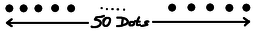A 50 dot game
So let's start with a game of 50 dots.

This has a G-value of 5, so there must be a winning move. We try taking an end-point, but g(48)=4, so that's no good. Try taking the second from end, but g(47)=4, so that's also no good. OK, we need to take an interior point.

Taking an interior point removes 3 from consideration, so we look at pairs of numbers that add up to 47 and see if we can find two that have the same G-value:

• (1,46) : g(1)=1, g(46)=2, nope;
• (2,45) : g(2)=1, g(45)=2, nope;
• (3,44) : g(3)=2, g(44)=3, nope;
• (4,43) : g(4)=0, g(43)=3, nope;
• (5,42) : g(5)=3, g(42)=0, nope;
• (6,41) : g(6)=1, g(41)=1, we have a winning move!

So we take the eighth dot from the left end, and its neighbours, and that leaves 6 on the left, and 41 on the right, and each collection has a G-value of 1. We XOR those together, and we have a G-value of 0, and thus it's a P position.

And so we continue. Suppose our opponent takes the middle point of the larger collection, leaving position (6,19,19).

What we do is observe that (19,19) is a P position, so if we can convert (6) into a P position, we're set. And we do that by taking an endpoint from the 6, leaving (4), which is a P position. So now we have two P positions, and we play each as a separate game. Whatever our opponent does, we do the right thing in that game, greatly simplifying the calculations we have to perform.

Lest you think that everything is sorted, there is a book called "Unsolved problems in combinatorial games" by Richard J Nowakowski. In that, question number 2 asks if all games such as we've been describing are, in fact, periodic.

We just don't know.

Take a specific example such as the game "Officers", which is 0.6 using the Octal Game notation. We don't know if that's periodic, and as of November 2012, Achim Flammenkamp's list of octal games has explored up to $2^{47}$ positions without a proven solution.

We just don't know.

So there are still problems to explore, results to be found, papers to be written, and games to be analysed. I hope this has been an interesting introduction to Combinatorial Game Theory.

## Credits and References:

 My thanks to Adam Atkinson, Adam Townsend, and Rob Eastaway of Maths Inspiration for extensive and detailed comments that have made this discussion infinitely better. My thanks also to Rob for introducing me to this intriguing game.

 Mathematical Games, Scientific American, March 1962, reprinted in The Unexpected Hanging and Other Mathematical Diversions, by Martin Gardner, pp. 93ff

 <<<< Prev <<<< One Of My Favourite Puzzles : >>>> Next >>>> Disappearing Trains On Virgin ...

 You should follow me on twitter## Send us a comment ...

 You can send us a message here. It doesn't get published, it just sends us an email, and is an easy way to ask any questions, or make any comments, without having to send a separate email. So just fill in the boxes and then

 Your name : Email : Message :

# ContentsSuggest a change ( <-- What does this mean?) / Send me email